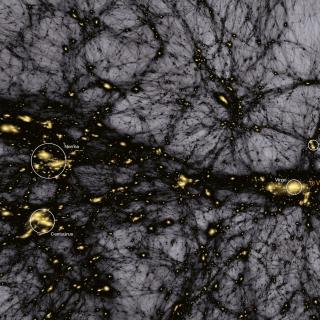# Density distribution of the cosmological matter field

Klypin, A.; Prada, F.; Betancort-Rijo, J.; Albareti, F. D.
Referencia bibliográfica

Monthly Notices of the Royal Astronomical Society, Volume 481, Issue 4, p.4588-4601

Fecha de publicación:
12
2018
Descripción
The one-point probability distribution function (PDF) of the matter density field in the universe is a fundamental property that plays an essential role in cosmology for estimates such as gravitational weak lensing, non-linear clustering, massive production of mock galaxy catalogues, and testing predictions of cosmological models. Here we make a comprehensive analysis of the dark matter PDF, using a suite of ˜7000 N-body simulations that covers a wide range of numerical and cosmological parameters. We find that the PDF has a simple shape: it declines with density as a power-law P ∝ ρ-2, which is exponentially suppressed on both small and large densities. The proposed double-exponential approximation provides an accurate fit to all our N-body results for small filtering scales R < 5 h-1 Mpc with rms density fluctuations σ > 1. In combination with the spherical infall model that works well for small fluctuations σ < 1, the PDF is now approximated with just few per cent errors over the range of 12 orders of magnitude - a remarkable example of precision cosmology. We find that at {˜ } 5{-}10{{ per cent}} level the PDF explicitly depends on redshift (at fixed σ) and on cosmological density parameter Ωm. We test different existing analytical approximations and find that the often-used lognormal approximation is always 3-5 times less accurate than either the double-exponential approximation or the spherical infall model.